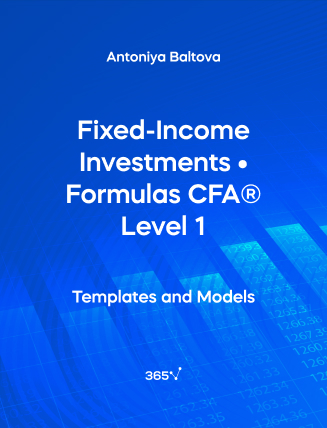## Fixed-Income Investments • Formulas CFA® Level 1###### Antoniya Baltova

Need an all-in-one list with the Fixed-Income Investments formulas included in the CFA Level 1 Exam? We have compiled them for you here. The relevant formulas have been organized and presented by chapter. In this section, we will cover the following topics — Fixed-Income Securities and Fixed-Income Valuation.

## 1. Fixed-income Securities

Conversion ratio

Conversion~ratio = \frac {Par~value}{Conversion~price}

Conversion value

Conversion~value = Share~price \times Conversion~ratio

Conversion ~premium/discount=Convertible~bond~price - Conversion~value

## 2. Introduction to Fixed-income Valuation

Fixed-rate bonds

PV = \frac {PMT}{(1 + r)^1} + \frac {PMT}{(1 + r)^2}+…+ \frac {PMT+FV}{(1 + r)^N}

PV = Present value (price)
PMT = Coupon payment amount per period
r = Discount rate
N = Number of periods to maturity
FV = Face value/par value/future value

PV = \frac {PMT}{(1 + Z_1)^1} + \frac {PMT}{(1 + Z_2)^2}+…+ \frac {PMT+FV}{(1 + Z_N)^N}

PV = Present value (price)
PMT = Coupon payment amount per period
Z_n = Spot rate per period
N = Number of periods to maturity
FV = Face value/par value/future value

PVFlat = PVFull - AI
PVFull = \Bigg [\frac {PMT}{(1 + r)^{1-\frac {t}{T}}} + \frac {PMT}{(1 + r)^{2-\frac {t}{T}}}+…+ \frac {PMT}{(1 + r)^{N-\frac {t}{T}}} \Bigg ]
PVFull = PV \times (1 + r)^{\frac {t}{T}}
AI = \frac {t}{T} \times PMT

PVFull = Full price of a bond
PVFlat = Flat price of a bond
AI = Accrued interest
PMT = Coupon payment amount per period
N = Number of periods to maturity
T = Number of days within a coupon payment period
t = Number of days from the last coupon payment to the settlement date

Fixed-rate bonds

\bigg( 1+\frac {APR_m}{m}\bigg)^m=\bigg( 1+\frac {APR_n}{n}\bigg)^n

APRm = Annual percentage rate for m
m = Periodicity that you are converting from
APRn = Annual percentage rate for n
n = Periodicity that you are converting to

Current yield

Current~yield = \frac {Total~PMT~in~a~year}{Flat~Price}

Floating Rate Notes (FRNs)

PV=\frac { \frac {(Index + QM) \times FV}{m} }{ \Big(1+\frac {Index + DM}{m} \Big)^1} + \frac { \frac {(Index + QM) \times FV}{m} }{ \Big(1+\frac {Index + DM}{m} \Big)^2}+…+ \frac { \frac {(Index + QM) \times FV}{m}+FV }{ \Big(1+\frac {Index + DM}{m} \Big)^N}

PV = Present value (price) of a floating-rate note
Index = Reference rate (stated as an annual percentage rate)
QM = Quoted margin (stated as an annual percentage rate)
FV = Future value paid at maturity (par value)
m = Periodicity of the floating- rate note, or the number of payment periods per year
DM = Discount/required margin (stated as an annual percentage rate)
N = Number of evenly spaced periods to maturity

Money market instruments

PV = FV \times \bigg ( 1-\frac {Days}{Year} \times DR \bigg )
FV = PV + \bigg ( PV \times \frac {180}{365} \times AOR \bigg )

PV = Present value (price) of the money market instrument
FV = Future value (face/par value) of the money market instrument
Days = Number of days between settlement and maturity
Year = Number of days in the year
DR = Discount rate (stated as an annual percentage rate)
AOR = Add-on rate (stated as an annual percentage rate)

Forward rates

(1 + Z_A)^A \times (1 + IFR_{A,B-A})^{B-A} = (1 + Z_B)^B

Z_n = Spot rate
IFR = Implied forward rate

Follow the links to find more formulas on Quantitative Methods, Economics, Corporate Finance, Alternative Investments, Financial Reporting and Analysis, Portfolio Management, Equity Investments, and Derivatives, included in the CFA® Level 1 Exam.Top